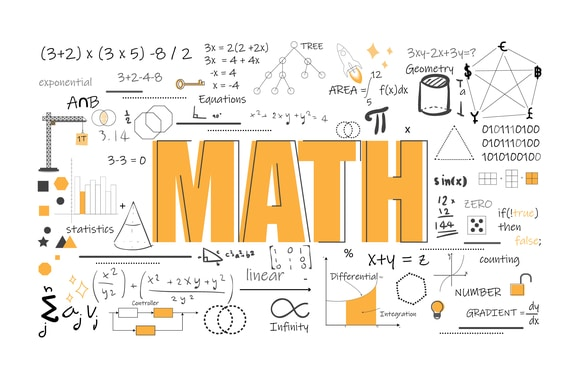# Factorization

Welcome to Class !!

In today’s Mathematics class, We will be looking at Factorisation. We hope you enjoy the class!### FACTORISATION

CONTENT

• Factorization of simple expression
• The difference of two squares
• Factorization of a quadratic expression

##### FACTORISATION OF SIMPLE EXPRESSION

To factorize an expression completely, take the HCF outside the bracket and then divide each term with the HCF.

Example:

Factorize the following completely.

1. 8xy + 4x2y
2. 6ab – 8a2b + 12ab

Solution:

1. 8xy + 4x2y

8xy = 2 X 2 X 2 X x X y

4x2y = 2 X 2 X xXxX y

HCF = 4xy

8xy + 4x2y = 4xy ( 2 + x)

= 4xy ( 2 + x)

1. 9a2bc3 – 12ab2c2

9a2bc3 = 3 X 3 X a X a X c X c X c

12ab2c2 = 2 X 2 X 3 X b X b X c X c

HCF = 3abc2

= 3abc2 (3ac – 4b)

EVALUATION

factorize the following expression

1. 9x2yz2 – 12x3z3
2. 14cd + 35cd2f
3. 20m2n – 15mn2

##### FACTORISATION BY GROUPING

To factorize an expression containing four terms, you need to group the terms into pairs. Then factorize each pair of terns.

Example:

factorize ab – 2cb + 2cf – af

Solution:

Group ab and af together and 2cb and 2cf together

i.e. ab – 2cb + 2cf –af = ab – af – 2cb + 2cf

= a( b – f ) -2c( b – f )

= (a – 2c)( b – f)

EVALUATION

factorize these expressions;

1. 16uv – 12vt + 20mu – 15mt
2. ap +aq +bq + bp
3. mn – pq-pn +mq

A quadratic expression has two (2) as its highest power; hence this at times is called a polynomial of the second order. The general representation of quadratic expression is

ax 2 + bx + c where a ≠ 0.

From the above expression, a, b, and c stands for a number and are called constants.

NOTE

1. if ax 2 +bx + c= 0, this is known as quadratic equation
2. a is coefficient of x2, b is coefficient of x and c is a constant term.
3. When an expression contains three terms, it is known as trinomial.
4. To be able to factorize trinomial, we need to convert it to contain four terms.

Examples: factorization of trinomial of the form x2 +bx + c.

1. Factorise x2 +7x +6

Steps:

• Multiply the 1st and the last term (3rd term) of the expression.
• Find two factors of the above multiple such that if added gives the second term (middle) and when multiplied gives the result in step 1.
• Replace the middle term with these two numbers and factorize by grouping.

Solution to example:

X2 x 6 = 6x2

Factors: 6 and 1

X2 + 6x + x + 6

X(x+6) +1(x+6)

(x+6)(x+1)

EVALUATION

1. z2 – 2z + 1
2. x2 +10x – 24

#### FACTORISATION OF QUADRATIC EQUATIONS OF THE FORM ax 2 +bx +c

Example:  5x 2 -9x +4

Solution:

Product: 5x 2 x 4 = 20x 2

Factors: -5 and -4

Sum: -5-4 = -9

Hence, 5x 2 – 9x + 4

5x2  -5x -4x +4

5x(x-1)-4(x-1)

(5x-4)(x-1)

EVALUATION

1. 2x 2 +13x +6
2. 13d 2 – 11d – 2

###### FACTORISATION OF TWO SQUARES

To factorise two squares with a difference, we need to remember the law guiding difference of two squares i.e. x 2 – y2  = (x + y) (x- y).

Examples:

1. P 2 – Q2 = (P+Q) (P-Q)
2. 36y 2 – 1= 6 2 y 2 –  1 2

= (6y)2  – 1 = ( 6y+1) (6y-1).

EVALUATION

1. 121- y 2
2. x2y2 – 42

Essential Mathematics for J.S.S.3 Pg29-36

Exam focus for J.S.S CE Pg101-105-

WEEKEND ASSIGNMENT

1. The coefficient of x 2 in x 2  + 3x -5 is  3 B. 1 C. -5 D. 2
2. Simplify
• A. e 2 – f 2 (e+f)(e-f)
• B. (e+f)(f+e)
• C. (e-f)(f-e) D. e+f
3. Factorize x 2 +x -6 (x+3)(x+2) B. (x-2)(x+3) C. (x+1)(x+5) D. x + 2
4. Solve by grouping 5h 2 -20h + h – 4 A. (h-4)(5h+1) B. (h+4)(5h-1) C. (h+2)(h-5) D. h – 4
5. 49m 2 – 64n 2 when factorized will be A. (7m+8n)(8m+7n) B. (8m-7n)(8m+7n)
6. (7m-8n)(7m+8n) D. 7m – 8n

THEORY

Factorise the following

1. 4p2 – 12p +9q2
2. f 2 – 2f + 1

We have come to the end of this class. We do hope you enjoyed the class?

Should you have any further question, feel free to ask in the comment section below and trust us to respond as soon as possible.

In our next class, we will be talking about Simple Fractional Expressions. We are very much eager to meet you there.

Are you a Parent? Share your quick opinion and win free 2-month Premium Subscription

### 1 thought on “Factorization”

1.I don’t understand the examples in factorization of two squares

Don`t copy text!